Next: 2.4 Phonon Transport Up: 2.3.2 Transmission Function Previous: 2.3.2 Transmission Function   Contents

### 2.3.2.1 Surface Green's Function

To calculate the properties of the channel, we assume semi-infinite contacts. Using the NEGF formalism, these contacts yield a finite surface energy [32,55]. The channel couples with only a finite number of surface atoms in the contacts that contain, however, the information of entire semi-infinite lead. Therefore, since most of the elements in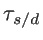are zero, the matrices can be reduced to smaller ones,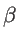and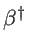, which only contain the couplings between neighboring unit cells. In this case, the first elements of the Hamiltonian that contain the information of a unit cell,, is used to describe the first section/unit cell of the contact, whereas the rest of the contacts can be modeled by self-energy matrices. Due to the periodicity of the semi-infinite contacts, the surface Green's function can be calculated iteratively as: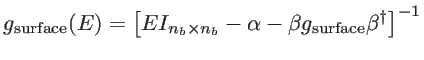(2.50)

where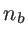is the number of orbital bases in the unit cell. The size of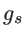can be further reduced to the number of surface atoms in the contact that couple to the channel atoms, and not the atoms of the entire unit cell, which simplifies computation significantly. To solve the non-linear matrix equation 2.50 different algorithms have been proposed . One of the most efficient one is the Sancho-Rubio method . After obtaining the surface Green's function, one can easily calculate the corresponding self-energy matrix as: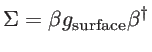(2.51)Next: 2.4 Phonon Transport Up: 2.3.2 Transmission Function Previous: 2.3.2 Transmission Function   Contents
H. Karamitaheri: Thermal and Thermoelectric Properties of Nanostructures